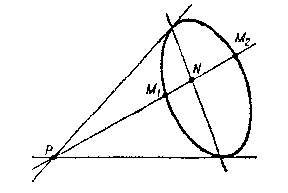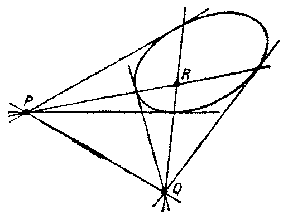# Polar

## Polar of a point with respect to a conic

The polar of a point $P$ with respect to a non-degenerate conic is the line containing all points harmonically conjugate to $P$ with respect to the points $M _ {1}$ and $M _ {2}$ of intersection of the conic with secants through $P$( cf. Cross ratio). The point $P$ is called the pole. If the point $P$ lies outside the conic, then the polar passes through the points of contact of the two tangent lines that can be drawn through $P$( see Fig. a). If the point $P$ lies on the curve, then the polar is the tangent to the curve at this point. If the polar of the point $P$ passes through a point $Q$, then the polar of $Q$ passes through $P$( see Fig. b).Figure: p073400aFigure: p073400b

Every non-degenerate conic determines a bijection between the set of points of the projective plane and the set of its straight lines, which is a polarity (a polar transformation). Figures that correspond under this transformation are called mutually polar. A figure coinciding with its polar figure is called autopolar, or self-polar (see, for example, the self-polar triangle $PQR$ in Fig. b).

Analogously one defines the polar (polar plane) of a point with respect to a non-degenerate surface of the second order.

The concept of a polar relative to a conic can be generalized to curves of order $n$. Here, a given point of the plane is put into correspondence with $n - 1$ polars with respect to the curve. The first of these polars is a curve of order $n - 1$, the second, which is the polar of the given point relative to the first polar, has order $n - 2$, etc., and, finally, the $( n - 1 )$- st polar is a straight line.

How to Cite This Entry:
Polar. Encyclopedia of Mathematics. URL: http://encyclopediaofmath.org/index.php?title=Polar&oldid=48225
This article was adapted from an original article by A.B. Ivanov (originator), which appeared in Encyclopedia of Mathematics - ISBN 1402006098. See original article